# Climbing Stairs Problem

Key takeaway: an excellent counting problem to learn problem-solving using dynamic programming and application of the Fibonacci series in problem-solving. One can find a lot of similar DP problems asked during the coding interview.

### Let’s understand the problem

There is a staircase of n steps and you can climb either 1 or 2 steps at a time. We need to count and return the total number of unique ways to climb the top of the staircase. The order of steps matters.

Example 1

Input: n = 3, Output: 3

Explanation: There are 3 unique ways of climbing the staircase: [1,1,1], [2,1] and [1,2]

Example 2

Input: n = 5, Output: 8

Explanation: There are 8 unique ways of climbing the staircase: [1,1,1,1,1], [1,1,1,2], [1,1,2,1], [1,2,1,1], [2,1,1,1], [1,2,2], [2,1,2], [2,2,1]

### Discussed solution approaches

• The brute force approach using a recursive approach
• Using the bottom-up approach of dynamic programming
• Using the idea of the Fibonacci sequence
• Using Binet’s method of matrix exponentiation

### The brute force approach  using a recursive approach

Solution Idea

This is a counting problem where we need to count all unique ways to reach the nth stair, but the critical question is: how do we count all the possibilities?

We have two different choices at the start: either climb the 1st stair or climb the 2nd stair because we can only jump 1 or 2 steps at a time.

• If we take one step from the ground, then the smaller sub-problem = climbing the nth stair from the 1st stair.
• If we take the two-step from the ground, then the smaller sub-problem = climbing the nth stair from the 2nd stair.
• So we can solve the given counting problem recursively by adding the solution of the sub-problems: climbStairs(0, n) = climbStairs(1, n) + climbStairs(2, n)

We can also think with another analogy: we can reach the nth stair from (n-1)th stair or the (n-2)th stair by taking 1 and 2 steps, respectively. So total number of ways to reach nth stair = Total number of ways to reach (n-1)th stair + Total number of ways to reach (n-2)th stair => climbStairs(n) = climbStairs(n-1) + climbStairs(n-2)

Solution Pseudocode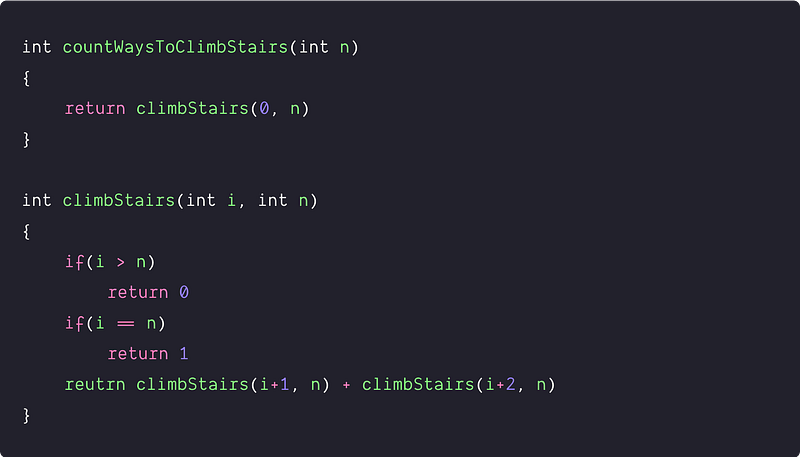Time and space complexity analysis

• Input size of the original problem = n, Input size of the 1st subproblem = n-1, input size of the 2nd sub-problem = n-2
• So we write the recurrence relation of the time complexity in terms of the input size i.e T(n) = T(n-1) + T(n-2), where T(1) = 1 and T(2) = 2
• The above function is similar to the recurrence relation of the Fibonacci sequence. So time complexity = O(2^n) (Think!)
• There is another rough analogy : we have two choices with each stair (excluding 0th and nth) i.e either we include it in the solution or exclude it. The total number of possibilities is ~ 2^n, so time complexity = O(2^n).
• But why the time complexity is exponential times? We can visualize it better using the recursion tree where are solving the same sub-problem again and again during the recursion. Here is a basic example for n = 4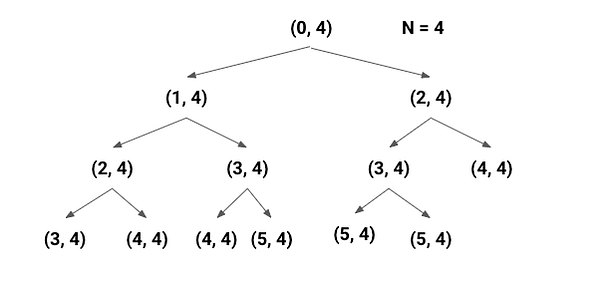Space Complexity = O(n), The depth of the recursion tree can go up to n and recursion will allocate call stack of size O(n). Think!

### Using the bottom-up approach of dynamic programming

Solution Idea and Steps

Since overlapping subproblems are present in this scenario, so we can optimize the solution using dynamic programming. Here we solve each sub-problem once, store the results in extra memory, and retrieve their results in O(1) instead of calculating again. We need to take care of the following steps to solve the problem using the bottom-up approach.

• Table size and structure : There is a total (n+1) of different subproblems with input sizes from 0 to n. So we need to take the stair[] table of size n+1 to store the solution to these sub-problems.
• Table Initialisation : Before building the solution in a bottom-up manner, we initialize the table by using the basic version of the solution. We can get this idea from the base case of the recursive solution. stair = 1, stair = 2.
• Iterative structure to fill the table : Now we define the iterative structure to fill the table or building the solution in a bottom-up manner. We can get this idea from the recursive structure of the recursive solution. Here is an insight: recursion is coming top-down and solving the larger problem via the solution of the smaller sub-problems. In a similar but reverse fashion, we need to go bottom-up and combine smaller problems to build the solution for the larger problem.

The total number of ways to reach the ith stair = Total number of ways to reach (i-1)th stair + Total number of ways to reach (i-2)th stair.

stair[i] = stair[i-1] + stair[i-2]

• Returning the final solution : After storing the results in the table, our final solution gets stored at the last Index of the array i.e. return stair[n].

Solution Pseudocode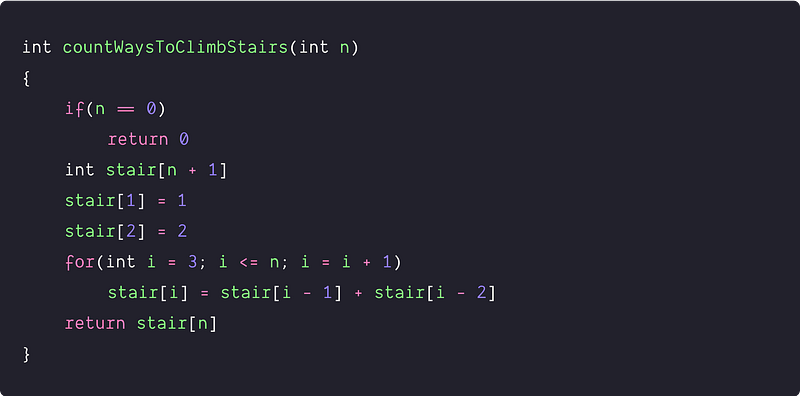Time and Space complexity analysis

We are running a single loop and doing constant operations at each step of the iteration, so time complexity = O(n). Space complexity = O(n), because we are using extra space of size n+1.

### Using the idea of the Fibonacci sequence

Solution Idea

If we observe the above approaches, the recursive and iterative structures are similar to the expression of the Fibonacci series. So rather than using recursion or using extra memory to store the solution of sub-problems, we can think to apply the in-place solution to find the nth number of the Fibonacci series, where 1 and 2 are the first and second term respectively.

fib(n) = fib(n-1) + fib(n-2), where fib(1) = 1 and fib(2) = 2

Solution Pseudocode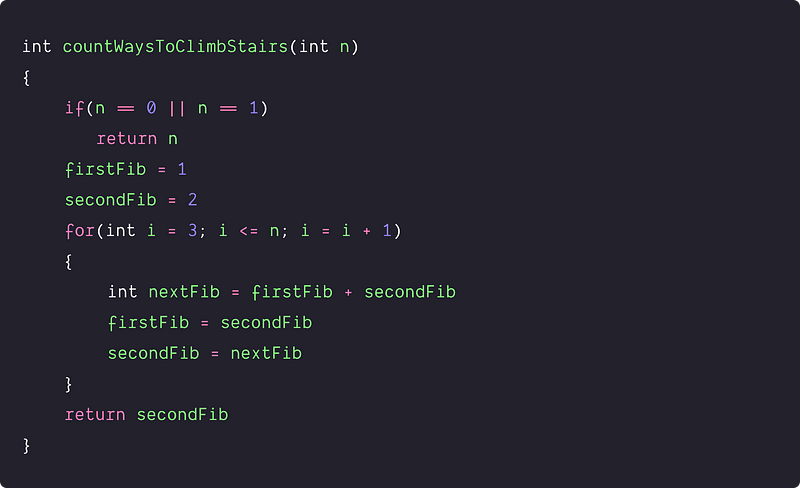Time and space complexity analysis

We are just running a single loop and doing constant operations at each step of the iteration, so time complexity = O(n), Space complexity = O(1), we are only using a constant number of variables.

### Using Binet’s method of matrix exponentiation

Solution Idea

This is an efficient method to find the nth Fibonacci number, which uses this idea :  if we multiply the matrix M = [[1,1],[1,0]] n times to itself or calculate power(M, n ), then we get the (n+1)th Fibonacci number as the element at 0th row and 0th column i.e. at the position (0, 0) in the final matrix.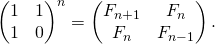We can apply the above method because the recurrence relation of this problem is similar to the Fibonacci sequence. The only change we need to do is to modify the base case to 1 and 2 instead of 0 and 1 in the Fibonacci series.

So, we can use the same initial matrix M and return the element at index (0,0) of the matrix M^n i.e. the (n+1)th Fibonacci number. We can do this because the nth term of the recurrence relation is equal to the (n+1) th Fibonacci number (Base cases are the 2nd and 3rd terms of the normal Fibonacci series).

Solution Pseudocode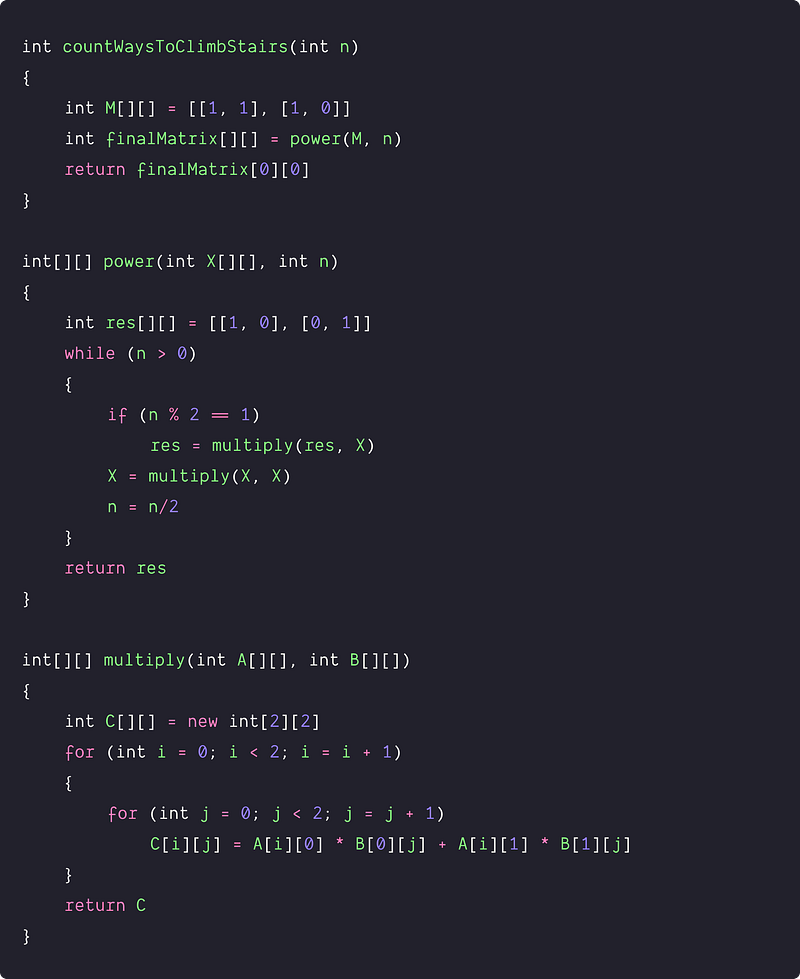Time and space complexity analysis

• The time complexity of 2x2 matrix multiplication = O(1) (Think!)
• Inside the power() function, while loop will run logn times, so the multiply() function has been called logn number of times. So time complexity = O(logn) * O(1) = O(logn)
• We are only using constant size extra matrix res for the calculation of the nth power of the matrix. So space complexity = O(1)

### Critical ideas to think!

• Can we solve this problem using any other approach?
• How do we modify the above algorithms if we can climb up to k steps at a time? What would be the recurrence relation for this?
• How will the solution change if the order of steps you take didn’t matter? In this case {1,1,2}, {1,2,1} and {2,1,1} will be counted as one solution.
• Explore the correctness proof of the matrix exponentiation approach.

### Comparison of time and space complexities

• Brute force recursive approach: Time = O(2^n), Space = O(n)
• Using bottom-up approach of DP: Time = O(n), Space = O(n)
• Using the Fibonacci sequence: Time = O(n), Space = O(1)
• Using the Binet’s method of matrix exponentiation: Time = O(logn), Space = O(1)

### Similar coding problems to practice

• Find the number of ways to climb N stairs by taking at most k leaps
• Find the nth Fibonacci Number
• Count the number of ways to reach the nth stair using steps 1, 2, or 3.
• Consider a game where a player can score 3 or 5 or 10 points in a move. Given a total score n, find the number of ways to reach the given score.
• Given n, count the number of ways to express n as a sum of 1, 3, and 4.

Enjoy learning, Enjoy algorithms!

Get well-designed application and interview centirc content on ds-algorithms, machine learning, system design and oops. Content will be delivered weekly.